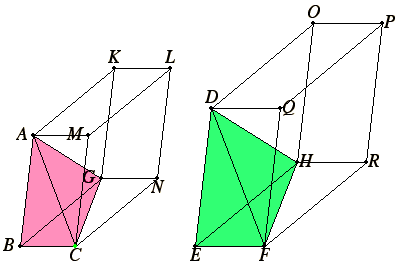# Proposition 8

Similar pyramids with triangular bases are in triplicate ratio of their corresponding sides.

Let there be similar and similarly situated pyramids with triangular bases AB and DEF vertices G and H.

I say that the pyramid ABCG has to the pyramid DEFH the ratio triplicate of that which BC has to EF.

Complete the parallelepipedal solids BGML and EHQP.Now, since the pyramid ABCG is similar to the pyramid DEFH, therefore the angle ABC equals the angle DEF, the angle GBC equals the angle HEF, the angle ABG equals the angle DEH, and AB is to DE as BC is to EF, and as BG is to EH.

And since AB is to DE as BC is to EF, and the sides are proportional about equal angles, therefore the parallelogram BM is similar to the parallelogram EQ. For the same reason BN is also similar to ER, and BR similar to EO.

XI.24

Therefore the three parallelograms MB, BK, and BN are similar to the three EQ, EO, and ER. But the three parallelograms MB, BK, and BN are equal and similar to their three opposites, and the three EQ, EO, and ER are equal and similar to their three opposites.

Therefore the solids BGML and EHQP are contained by similar planes equal in multitude. Therefore the solid BGML is similar to the solid EHQP.

XI.33

But similar parallelepipedal solids are in the triplicate ratio of their corresponding sides. Therefore the solid BGML has to the solid EHQP the ratio triplicate of that which the corresponding side BC has to the corresponding side EF.

But the solid BGML is to the solid EHQP as the pyramid ABCG is to the pyramid DEFH, for the pyramid is a sixth part of the solid, because the prism which is half of the parallelepipedal solid is also triple the pyramid. Therefore the pyramid ABCG has to the pyramid DEFH the ratio triplicate of that which BC has to EF.

Q.E.D.

# Corollary

From this it is clear that similar pyramids with polygonal bases are also to one another in the triplicate ratio of their corresponding sides.

For, if they are divided into the pyramids contained in them which have triangular bases, by virtue of the fact that the similar polygons forming their bases are also divided into similar triangles equal in multitude and corresponding to the wholes, then the one pyramid with a triangular base in the one complete pyramid is to the one pyramid with a triangular base in the other complete pyramid as all the pyramids with triangular bases contained in the one pyramid is to all the pyramids with triangular bases contained in the other pyramid, that is, the pyramid itself with a polygonal base, to the pyramid with a polygonal base.

But the pyramid with a triangular base is to the pyramid with a triangular base in the triplicate ratio of the corresponding sides, therefore also the pyramid with a polygonal base has to the pyramid with a similar base the ratio triplicate of that which the side has to the side.

Therefore, similar pyramids with triangular bases are in triplicate ratio of their corresponding sides.

Q.E.D.

## Guide

Heath gives a good argument that the corollary was added later.

#### Use of this proposition

This proposition is used to show similar cones are in triplicate ratio of the diameters of their bases in proposition XII.12. Also, the corollary justifies a statement in the corollary of XII.17 concerning similar solids.